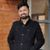## TyroCity is a community of amazing learners

We're a place where students share, stay up-to-date, learn and grow.

# Discussion on: How can a positive NPV end up with negative NPV with the same set of cash flow and time horizonAngel Paudel

Net present value (NPV) in financial term refers to the measurement of profit calculated by subtracting the present value of cash outflow which includes of the initial cost and further funneling of funds into the project from the present values of cash inflows over the set period of time (Ljubić, Mrša & Stanković, 2013).

As per the given criteria, to look into a project with positive Net Present Value (NPV) these details are taken into account/consideration:

Total initial investment: NRs. 5,00,000
Discount Rate: 5%
Timeframe: 5 years

Another assumption made for the calculation is that no further investment has been made within the five years taken into consideration. The cash flow is considered to be even and of NRs. 1,25,000 every year for five years consistently. The discount rate is considered to be fixed and consistent for every year taken into account. The value within brackets is to represent that they denote negative value.

Here’s the calculation for the NPV on the above set parameters:

Year Cash flow (CF) Present Value (PV) = [1/(1+i)^n] Discounted cash flow (CF*PV)
0 (500000) 1 (500000)
1 125000 0.95 118750
2 125000 0.91 113750
3 125000 0.86 107500
4 125000 0.82 102500
5 125000 0.78 97500
NPV 40000

In this case, we obtained a positive NPV which is NRs. 40,000. However, to end up with a negative NPV while using the same cash flow and time horizon, the discount rate should be varied. All the other parameters are considered to be the same except for the discount rate which is now considered to be 10% instead of 5%. Here’s the calculation with the updated discount rate:

Year Cash flow (CF) Present Value (PV) = [1/(1+i)^n] Discounted cash flow (CF*PV)
0 (500000) 1 (500000)
1 125000 0.91 113750
2 125000 0.83 103750
3 125000 0.75 93750
4 125000 0.68 85000
5 125000 0.62 77500
NPV (26250)

As we can see from the demonstration, with the change in the discount rate, we end up with a negative NPV of NRs. 26,250.

With these demonstrations, we can conclude that we can end up with negative NPV while keeping the same cash flow and time horizon with just change made to the discount rate.

References

Ljubić, D., Mrša, J., & Stanković, S. (2013). Interaction of Net Present Value, Cash Flow and Financial Statements. SSRN Electronic Journal , 1-2.